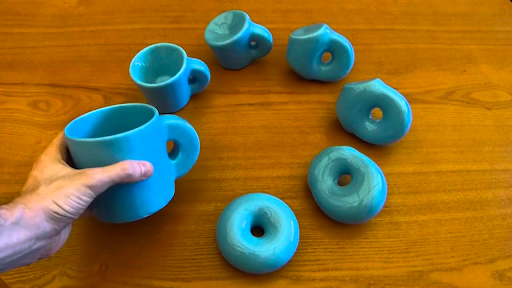top of page
Search

# So You Want to Learn Advanced Math?(Cover Artist: Sophie Cheng)

A Basic Landscape of Higher Mathematics

Let’s say you’re an aspiring math student and want to take that extra step to learn more advanced topics. You might think “Where would I go for a guide?” Well, lucky you! Here, I’ll try to provide a basic landscape of more advanced mathematics, beginning, of course, with the most notorious topics: calculus and linear algebra.

Calculus

The poster child of advanced mathematics is the one and only, calculus. Although this branch has a reputation for being difficult, don’t worry! Calculus isn’t the beast of mathematics that it’s sometimes made out to be. Put simply, calculus (more specifically, Calculus I and II) is the study of three operations: the limit, the derivative, and the integral. Put very simply, limits focus on the behavior of functions around a value rather than at that value, derivatives focus on the rate of change of functions at a given moment, and integrals focus on the area under functions.

Extensions of regular calculus include multivariable calculus, real analysis, and differential equations. While basic calculus is usually encountered at the later high school and early college level, you normally won’t see these extensions until a bit later in your college career. Multivariable calculus, as you might’ve already guessed, deals with extending calculus to multiple dimensions. Real analysis can be most simply understood as a more rigorous, proof-focused, and in-depth treatment of the calculus you would have learned up to that point. While real analysis might seem like just Calculus I and II but a bit harder from this description, real analysis truly is a whole different beast, and you’ll learn a ton of new theorems and strategies along the way. Differential equations are a lot more applied than some of the other math courses you can encounter later on; it focuses on how quantities change in relation to other quantities that change, with some examples being velocity and acceleration.

Linear Algebra

Linear algebra is another cornerstone of advanced mathematics. At a basic level, linear algebra focuses on two kinds of objects: vectors and matrices. Put simply, vectors are objects that have both a direction and a magnitude and matrices are arrays of numbers, symbols, and expressions. This amount of generality with the definition of vectors and matrices allows them to be very flexible in what phenomena they can model. Although vectors and matrices might seem on the more theoretical side in the beginning, they have a lot of applications to a vast array of fields, both inside and outside of math. They help with procedurally solving linear equations, optimization, linear regression, and much, much more. For example, linear algebra is very helpful in computer graphics, since vectors and matrices are a great tool for visualizing objects. In general, linear algebra forms a strong foundation for mathematics past linear algebra, such as geometric algebra.

Set Theory

Although you might’ve encountered some basic (naïve) set theory earlier in your education, it can be taken to a newer and more formal level. Set theory is really important not only because of how it shaped the development of mathematics in the 19th and 20th centuries (try Googling intuitionists vs. formalists), but also because of how it forms the basis of a lot of mathematics that came after it. In particular, the axiomatic systems developed from set theory are crucial as formal foundations for mathematics. Set theory also formalizes the concept of infinity and has been subject to quite a bit of controversy over which axioms should be considered valid. Furthermore, there are indeed some applications of set theory to fields like probability theory, computer science, and even philosophy.

TopologyTopology is an interesting subfield of mathematics; you may have heard the common joke that topologists consider donuts and mugs to be the same type of object because they both only contain one hole. However, topology is much more complex than what this joke makes it out to be. Topology is the study of objects and their properties under “continuous deformations.” This definition contrasts geometry, which is concerned with the exact lengths, angle measures, etc. of geometric figures. Topology is deeply related to set theory; in fact, they are so interlinked that a common course about the two is called “point-set topology.”

So, that ends my brief summary about the world of higher mathematics. I hope this information has been useful to you and guides you on further mathematical endeavors.